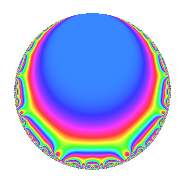# Properties

 Label 4029.2.a.cLevel 4029 Weight 2 Character orbit 4029.a Self dual yes Analytic conductor 32.172 Analytic rank 1 Dimension 2 CM no Inner twists 1

# Related objects

## Newspace parameters

 Level: $$N$$ = $$4029 = 3 \cdot 17 \cdot 79$$ Weight: $$k$$ = $$2$$ Character orbit: $$[\chi]$$ = 4029.a (trivial)

## Newform invariants

 Self dual: yes Analytic conductor: $$32.1717269744$$ Analytic rank: $$1$$ Dimension: $$2$$ Coefficient field: $$\Q(\sqrt{2})$$ Coefficient ring: $$\Z[a_1, a_2]$$ Coefficient ring index: $$1$$ Twist minimal: yes Fricke sign: $$1$$ Sato-Tate group: $\mathrm{SU}(2)$

## $q$-expansion

Coefficients of the $$q$$-expansion are expressed in terms of $$\beta = \sqrt{2}$$. We also show the integral $$q$$-expansion of the trace form.

 $$f(q)$$ $$=$$ $$q + \beta q^{2} + q^{3} + ( -1 + \beta ) q^{5} + \beta q^{6} + q^{7} -2 \beta q^{8} + q^{9} +O(q^{10})$$ $$q + \beta q^{2} + q^{3} + ( -1 + \beta ) q^{5} + \beta q^{6} + q^{7} -2 \beta q^{8} + q^{9} + ( 2 - \beta ) q^{10} -4 \beta q^{11} + \beta q^{14} + ( -1 + \beta ) q^{15} -4 q^{16} + q^{17} + \beta q^{18} + ( -5 + 2 \beta ) q^{19} + q^{21} -8 q^{22} + ( -3 + 3 \beta ) q^{23} -2 \beta q^{24} + ( -2 - 2 \beta ) q^{25} + q^{27} + ( 2 + 2 \beta ) q^{29} + ( 2 - \beta ) q^{30} + ( 4 - 4 \beta ) q^{31} -4 \beta q^{33} + \beta q^{34} + ( -1 + \beta ) q^{35} + ( -5 + 4 \beta ) q^{37} + ( 4 - 5 \beta ) q^{38} + ( -4 + 2 \beta ) q^{40} + ( 2 + 4 \beta ) q^{41} + \beta q^{42} + ( 2 - 6 \beta ) q^{43} + ( -1 + \beta ) q^{45} + ( 6 - 3 \beta ) q^{46} + ( -3 - 5 \beta ) q^{47} -4 q^{48} -6 q^{49} + ( -4 - 2 \beta ) q^{50} + q^{51} + ( -11 - \beta ) q^{53} + \beta q^{54} + ( -8 + 4 \beta ) q^{55} -2 \beta q^{56} + ( -5 + 2 \beta ) q^{57} + ( 4 + 2 \beta ) q^{58} + ( -5 + \beta ) q^{59} + ( 5 - 4 \beta ) q^{61} + ( -8 + 4 \beta ) q^{62} + q^{63} + 8 q^{64} -8 q^{66} -10 q^{67} + ( -3 + 3 \beta ) q^{69} + ( 2 - \beta ) q^{70} + ( -6 - 5 \beta ) q^{71} -2 \beta q^{72} + ( 2 - 9 \beta ) q^{73} + ( 8 - 5 \beta ) q^{74} + ( -2 - 2 \beta ) q^{75} -4 \beta q^{77} - q^{79} + ( 4 - 4 \beta ) q^{80} + q^{81} + ( 8 + 2 \beta ) q^{82} + ( 4 + \beta ) q^{83} + ( -1 + \beta ) q^{85} + ( -12 + 2 \beta ) q^{86} + ( 2 + 2 \beta ) q^{87} + 16 q^{88} + ( -12 + 4 \beta ) q^{89} + ( 2 - \beta ) q^{90} + ( 4 - 4 \beta ) q^{93} + ( -10 - 3 \beta ) q^{94} + ( 9 - 7 \beta ) q^{95} + ( 16 + \beta ) q^{97} -6 \beta q^{98} -4 \beta q^{99} +O(q^{100})$$ $$\operatorname{Tr}(f)(q)$$ $$=$$ $$2q + 2q^{3} - 2q^{5} + 2q^{7} + 2q^{9} + O(q^{10})$$ $$2q + 2q^{3} - 2q^{5} + 2q^{7} + 2q^{9} + 4q^{10} - 2q^{15} - 8q^{16} + 2q^{17} - 10q^{19} + 2q^{21} - 16q^{22} - 6q^{23} - 4q^{25} + 2q^{27} + 4q^{29} + 4q^{30} + 8q^{31} - 2q^{35} - 10q^{37} + 8q^{38} - 8q^{40} + 4q^{41} + 4q^{43} - 2q^{45} + 12q^{46} - 6q^{47} - 8q^{48} - 12q^{49} - 8q^{50} + 2q^{51} - 22q^{53} - 16q^{55} - 10q^{57} + 8q^{58} - 10q^{59} + 10q^{61} - 16q^{62} + 2q^{63} + 16q^{64} - 16q^{66} - 20q^{67} - 6q^{69} + 4q^{70} - 12q^{71} + 4q^{73} + 16q^{74} - 4q^{75} - 2q^{79} + 8q^{80} + 2q^{81} + 16q^{82} + 8q^{83} - 2q^{85} - 24q^{86} + 4q^{87} + 32q^{88} - 24q^{89} + 4q^{90} + 8q^{93} - 20q^{94} + 18q^{95} + 32q^{97} + O(q^{100})$$

## Embeddings

For each embedding $$\iota_m$$ of the coefficient field, the values $$\iota_m(a_n)$$ are shown below.

For more information on an embedded modular form you can click on its label.

Label $$\iota_m(\nu)$$ $$a_{2}$$ $$a_{3}$$ $$a_{4}$$ $$a_{5}$$ $$a_{6}$$ $$a_{7}$$ $$a_{8}$$ $$a_{9}$$ $$a_{10}$$
1.1
 −1.41421 1.41421
−1.41421 1.00000 0 −2.41421 −1.41421 1.00000 2.82843 1.00000 3.41421
1.2 1.41421 1.00000 0 0.414214 1.41421 1.00000 −2.82843 1.00000 0.585786
 $$n$$: e.g. 2-40 or 990-1000 Significant digits: Format: Complex embeddings Normalized embeddings Satake parameters Satake angles

## Inner twists

This newform does not admit any (nontrivial) inner twists.

## Twists

By twisting character orbit
Char Parity Ord Mult Type Twist Min Dim
1.a even 1 1 trivial 4029.2.a.c 2

By twisted newform orbit
Twist Min Dim Char Parity Ord Mult Type
4029.2.a.c 2 1.a even 1 1 trivial

## Atkin-Lehner signs

$$p$$ Sign
$$3$$ $$-1$$
$$17$$ $$-1$$
$$79$$ $$1$$

## Hecke kernels

This newform subspace can be constructed as the intersection of the kernels of the following linear operators acting on $$S_{2}^{\mathrm{new}}(\Gamma_0(4029))$$:

 $$T_{2}^{2} - 2$$ $$T_{5}^{2} + 2 T_{5} - 1$$